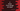# C program to check if two strings are equal or not### C program to check if two strings are equal or not :

In this tutorial, we will learn how to check if two strings are equal or not. We will ask the user to input both strings and then we will compare word by word . We will use one loop to iterate through the characters. You can use for loop to iterate, but in this example we will use while loop. Let’s take a look into the program :

### C program :

``````#include <stdio.h>
#include <stdlib.h>

int main()
{
//1
char *str1;
char *str2;

//2
int isEqual = 1;

//3
str1 = malloc(sizeof(char) * 255);
str2 = malloc(sizeof(char) * 255);

//4
printf("Enter the first string : ");
scanf("%[^\n]%*c", str1);

//5
printf("Enter the second string : ");
scanf("%[^\n]%*c", str2);

//6
int i = 0, j = 0;

//7
while (str1[i] != '\0' || str2[j] != '\0')
{
//8
if (str1[i] != str2[j])
{
isEqual = 0;
break;
}
//9
i++;
j++;
}

//10
if (isEqual == 0)
{
printf("Strings are not equal.");
}
else
{
printf("Both strings are equal.");
}
}``````

### Explanation :

The commented numbers in the above program denotes the steps number below :

1. Create two character pointer variables to store the first and the second string.
2. Create one integer variable isEqual and assign it a value 1. 1 means both strings are equal and 0 means both strings are not equal.
3. Before storing any values in the char * variables, we need to allocate memory . Allocate memory of 255 characters for both variables.
4. Ask the user to enter the first string. Store the string in the variable str1 .
5. Similarly, read the second string and store it in str2.
6. Initialize two integer variables i and j as 0.
7. Run one while loop and scan both strings one by one character. i denotes the current character for str1 and j denotes current character for str2.
8. If two characters in both the strings are not equal, set value of isEqual to 0 and break the loop.
9. If not equal, increate both i and j values.
10. Finally check if isEqual is 0 or not. If it’s value is 0 , both strings are equal and otherwise not.

### Sample Output :

``````Enter the first string : First string
Enter the second string : Second string
Strings are not equal.

Enter the first string : hello world
Enter the second string : hello world
Both strings are equal.

Enter the first string : HellO
Enter the second string : Hello
Strings are not equal.
``````